# How Uncertain Are Your Random Forest Predictions?

#### 2017-08-29

This vignette demonstrates how to use the surfin R package to compute uncertainty for random forest predictions. As noted by Wager et al., two sources of variability contribute to the variance of a random forest’s predictions: sampling variability (that the data we see is merely a sample from the unseen population) and Monte Carlo noise (that only a finite number of bagged replicates - i.e. the bootstrapped samples or subsamples trees are trained on - is used). We will compare two ways to estimate this variance: (1) a U-statistics based estimate (Mentch & Hooker 2016) based on subsampled trees that is implemented in this package (2) an infinitesimal jackknife based estimate (Wager, Hastie, Efron, 2014) based on bootstrapped trees using the R package randomForestCI provided by the authors. For further discussion of the differences between these first two variance estimates, see Section 2.1 of Mentch et al..

Categorical predictors are currently converted to their numeric equivalents, not made into indicator variables. This feature is pending. If you have categorical predictors, for now, make them indicators before calling the forest function.

This package is actively under development. Feedback, bug reports, etc. are very much welcome! Find our contact info on the package website.

## Setup

Let’s get started! First, load packages needed for this example:

library(surfin)
library(devtools)  # to install randomForestCI package from github
library(randomForest)  # to compare forest implementations
library(rpart) # for kyphosis data
library(MASS) # for Boston housing and breast cancer data

Next, install and load the randomForestCI R package:

#install_github("swager/randomForestCI")
library(randomForestCI)
library(grf)

## Regression

data(Boston)
x = Boston[,1:(ncol(Boston)-1)]
y = Boston[,ncol(Boston)]
#x = cu.summary[,c("Price","Country","Reliability","Type")]
#y = cu.summary$Mileage keep = !is.na(y) y = y[keep] x = x[keep,] keep = !apply(is.na(x),1,any) y = y[keep] x = x[keep,] n = length(y) train = sample(1:n,n*0.7) test = setdiff(1:n,train) xtrain = x[train,] ytrain = y[train] xtest = x[test,] ytest = y[test] Typical random forest implementations use bootstrapped trees. The U-statistics based variance estimate is based on subsamples which allows the Central Limit Theorem to be applied. The number of observations subsampled should be on the order of $$\sqrt{n}$$ where $$n$$ is the number of observations in the data set. Other parameters of interest are the number of trees, and B (the number of common observations between trees), and L (the number of trees sharing a observation). ntree, B and L are connected: if we use ntree=5000 trees and B=25 common observations between trees, L=5000/25 = 200 trees will share an observation, then the next 200 trees with share another observation, and so forth. So two of these three parameters need to be specified, and the third will automatically follow. Mentch & Hooker found in their experiments that a range of 10 to 50 for B works well empirically. Below, the variance option “ustat” automatically sets sampling to be performed without replacement with the appropriate subsample size. We specified ntree to be 5000 and B to be 25, so L follows as 200. fit = forest(xtrain,ytrain,var.type="ustat",B=25,ntree=5000) ##  "U-statistics based variance needs replace=FALSE. Forest was built with this option." ##  "U-statistics based variance needs individualTrees=TRUE. Forest was built with this option." Check out what the forest object outputs: names(fit) ##  "inbag.times" "oob.times" "forest" ##  "type" "varNames" "replace" ##  "var.type" "B" "sampSize" ##  "ntree" "individualTrees" "predicted" ##  "predictedAll" "predictedOOB" ### Prediction There are a variety of prediction options to choose from: 1. On the train set using only out-of-bag train observations 2. On the train set using all train observations 3. On the test set using all test observations u_train_oob = fit$predicted        # Case (1)
u_train = predict(fit,xtrain)  # Case (2)
temp = predict(fit,xtest,individualTrees=T)   # Case (3)
u_test = temp$predicted u_test_all = temp$predictedAll
temp = data.frame(u_train_oob,u_train)
head(temp)
##   u_train_oob  u_train
## 1    17.94845 17.21274
## 2    25.59756 22.86480
## 3    32.95932 32.50851
## 4    23.30036 23.09435
## 5     9.93262 10.00375
## 6    18.10525 16.71734
head(u_test)
##  21.73023 17.86578 20.02137 20.14602 19.96073 21.84161

### U-statistics based variance estimate

We can calculate and plot the u-statistics based variance estimate on the test set (Case (2)):

ustat = forest.varU(u_test_all,fit)
head(ustat)
##      y.hat  var.hat
## 1 21.73023 2.800562
## 2 17.86578 3.113678
## 3 20.02137 3.364887
## 4 20.14602 0.959694
## 5 19.96073 1.133617
## 6 21.84161 1.610932
plot(ustat)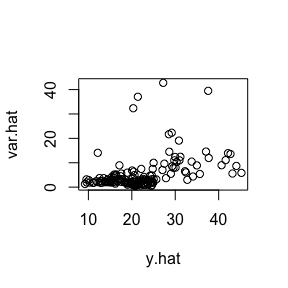It’s also possible to retrieve not just the variance, but also covariance between predictions:

temp = forest.varU(u_test_all,fit,covariance=TRUE)
y.hat = temp[]
cov = temp[]
dim(cov)
##  152 152
cov[1:6,1:6]
##           [,1]       [,2]       [,3]      [,4]       [,5]       [,6]
## [1,] 2.8005616  1.3798763 2.35868396 0.2858902 0.37539330  0.4766694
## [2,] 1.3798763  3.1136784 0.58256656 0.3287284 0.30679037 -0.1954635
## [3,] 2.3586840  0.5825666 3.36488742 0.0885935 0.07325777  0.6401547
## [4,] 0.2858902  0.3287284 0.08859350 0.9596940 0.96595207  0.3806474
## [5,] 0.3753933  0.3067904 0.07325777 0.9659521 1.13361688  0.3877370
## [6,] 0.4766694 -0.1954635 0.64015469 0.3806474 0.38773703  1.6109323

The (i,j) element in the covariance matrix represents the estimated covariance between observation i and observation j’s predictions.

The diagonals of the covariance matrix are exactly the variances we saw above:

unique(diag(cov) - ustat[,2])
##  0

We can also calculate variance estimate on the train set (Case (1)):

ij2 = forest.varIJ(fit$predictedAll,fit) head(ij2) ## y.hat var.hat ## 1 16.416135 0.8584885 ## 2 19.563145 8.8300243 ## 3 31.412246 0.7337753 ## 4 22.602270 0.5210426 ## 5 9.999253 0.6110645 ## 6 15.877614 9.3014199 plot(ij2)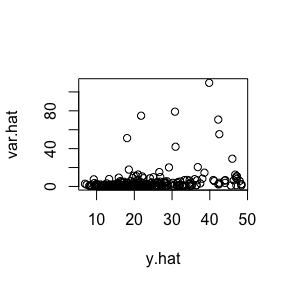### Compare to randomForest package Let’s compare our forest’s predictions to the predictions of the randomForest package: rf_train_oob = rf$predicted
plot(ij2_train_oob,rf_train_oob)
lines(ij2_train_oob,ij2_train_oob,lty="dashed")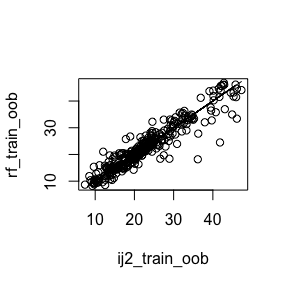### Infinitesimal jackknife based variance estimate on subsamples

fit = regression_forest(as.matrix(xtrain),ytrain,num.trees=5000)
tmp = predict(fit,xtrain,estimate.variance = TRUE)
ij_s = data.frame(tmp$predictions,tmp$variance.estimates)
head(ij_s)
##   tmp.predictions tmp.variance.estimates
## 1       16.457457              0.5017414
## 2       23.620753              4.3819401
## 3       30.255019              5.6906871
## 4       24.162986              1.1405607
## 5        9.757034              0.4234434
## 6       14.619335              0.3782159
plot(ij_s)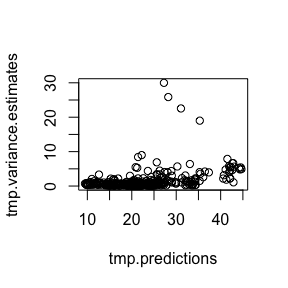### Combining the three estimates

We can look at the two components of the U-statistics based variance separately, to obtain a more equitable comparison with the infinitesimal jackknife based variance:

fit = forest(xtrain,ytrain,var.type="ustat",B=25,ntree=5000)
##  "U-statistics based variance needs replace=FALSE. Forest was built with this option."
##  "U-statistics based variance needs individualTrees=TRUE. Forest was built with this option."
ustat = forest.varU(fit$predictedAll,fit,separate=TRUE) head(ustat) ## y.hat var.hat var.hat.component1 var.hat.component2 ## 1 17.040441 9.026232 9.018845 0.007387001 ## 2 22.756579 4.141804 4.135040 0.006763889 ## 3 32.582935 6.487118 6.478309 0.008808755 ## 4 23.117471 1.226573 1.224871 0.001702090 ## 5 9.941546 1.976457 1.973618 0.002839079 ## 6 16.640265 19.442555 19.423059 0.019495677 head(ij) ## y.hat var.hat ## 127 16.53159 0.4033264 ## 343 20.20229 8.6657229 ## 264 31.06317 0.3073395 ## 91 23.06674 0.5220697 ## 387 9.35698 0.5545347 ## 143 14.84383 1.6900509 head(ij_s) ## tmp.predictions tmp.variance.estimates ## 1 16.457457 0.5017414 ## 2 23.620753 4.3819401 ## 3 30.255019 5.6906871 ## 4 24.162986 1.1405607 ## 5 9.757034 0.4234434 ## 6 14.619335 0.3782159 ### Sensitivity Analysis Let’s compare the variance estimates and see how they change when more trees are used in the forest. Like the examples above, B is set to 25. We vary the number of trees from 1000 to 7000: varU = vector("numeric") varIJ = vector("numeric") nts = seq(1000,7000,1000) for (nt in nts) { fit = forest(xtrain,ytrain,var.type="ustat",B=25,ntree=nt) varU = c(varU,mean(forest.varU(fit$predictedAll,fit)[,2]))
rf = randomForest(xtrain, ytrain, keep.inbag = TRUE, ntree=nt)
varIJ = c(varIJ,mean(randomForestInfJack(rf, xtrain, calibrate = TRUE)[,2]))
}
##  "U-statistics based variance needs replace=FALSE. Forest was built with this option."
##  "U-statistics based variance needs individualTrees=TRUE. Forest was built with this option."
##  "U-statistics based variance needs replace=FALSE. Forest was built with this option."
##  "U-statistics based variance needs individualTrees=TRUE. Forest was built with this option."
##  "U-statistics based variance needs replace=FALSE. Forest was built with this option."
##  "U-statistics based variance needs individualTrees=TRUE. Forest was built with this option."
##  "U-statistics based variance needs replace=FALSE. Forest was built with this option."
##  "U-statistics based variance needs individualTrees=TRUE. Forest was built with this option."
##  "U-statistics based variance needs replace=FALSE. Forest was built with this option."
##  "U-statistics based variance needs individualTrees=TRUE. Forest was built with this option."
##  "U-statistics based variance needs replace=FALSE. Forest was built with this option."
##  "U-statistics based variance needs individualTrees=TRUE. Forest was built with this option."
##  "U-statistics based variance needs replace=FALSE. Forest was built with this option."
##  "U-statistics based variance needs individualTrees=TRUE. Forest was built with this option."
plot(nts,varU,ylim=c(0,max(varU,varIJ)),cex.axis=0.6,ylab="Mean Est. Variance",xlab="Number of Trees",type="o",cex.lab=0.5)
points(nts,varIJ,col="blue",type="o")
legend("topright",legend=c("U-Stat","IJ"),col=c("black","blue"),lty="solid",cex=0.6)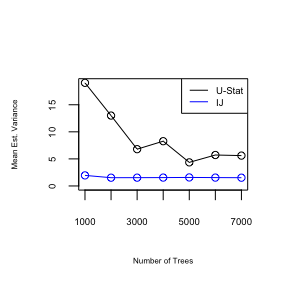print(varU)
##  19.047514 13.002373  6.795531  8.269921  4.357806  5.716746  5.608624
print(varIJ)
##  1.952063 1.525624 1.533387 1.546329 1.573048 1.544822 1.526463

We can also perform sensitivity analysis on the B parameter, the number of common observations between trees. Using 5000 trees:

varU = vector("numeric")
varIJ = vector("numeric")
bs = c(10,25,50,100)
for (b in bs)
{
fit = forest(xtrain,ytrain,var.type="ustat",B=b,ntree=5000)
varU = c(varU,mean(forest.varU(fit$predictedAll,fit)[,2])) } ##  "U-statistics based variance needs replace=FALSE. Forest was built with this option." ##  "U-statistics based variance needs individualTrees=TRUE. Forest was built with this option." ##  "U-statistics based variance needs replace=FALSE. Forest was built with this option." ##  "U-statistics based variance needs individualTrees=TRUE. Forest was built with this option." ##  "U-statistics based variance needs replace=FALSE. Forest was built with this option." ##  "U-statistics based variance needs individualTrees=TRUE. Forest was built with this option." ##  "U-statistics based variance needs replace=FALSE. Forest was built with this option." ##  "U-statistics based variance needs individualTrees=TRUE. Forest was built with this option." plot(bs,varU,ylim=c(0,max(varU,varIJ)),cex.axis=0.6,ylab="Mean Est. Variance",xlab="B",type="o",cex.lab=0.5)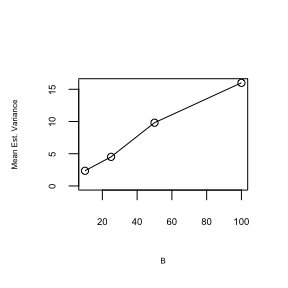print(varU) ##  2.353647 4.513786 9.814501 15.999577 Another parameter that’s important for the U-statistics based variance is the number of observations used by each tree. In a regular random forest this would just be $$n$$, the number of observations, but in a subsampled random forest we need to select the number of subsamples. Sensitivity analysis for this parameter pending. ## Binary Classification Next, we try classification. Currently only binary classification is supported: #data(biopsy) #x = biopsy[1:(ncol(biopsy)-1)] #y = biopsy[,ncol(biopsy)] x = kyphosis[,c("Age","Number","Start")] y = kyphosis$Kyphosis
keep = !is.na(y)
y = y[keep]
x = x[keep,]
keep = !apply(is.na(x),1,any)
y = y[keep]
x = x[keep,]
n = length(y)
train = sample(1:n,n*0.7)
test = setdiff(1:n,train)
xtrain = x[train,]
ytrain = y[train]
xtest = x[test,]
ytest = y[test]

The response is rather imbalanced:

table(y)
## y
##  absent present
##      64      17

### Prediction

Like for regression, we have several options for prediction.

fit = forest(xtrain,ytrain,var.type="ustat",B=50,ntree=5000)
##  "U-statistics based variance needs replace=FALSE. Forest was built with this option."
##  "U-statistics based variance needs individualTrees=TRUE. Forest was built with this option."
names(fit)
##   "inbag.times"      "oob.times"        "forest"
##   "type"             "key"              "varNames"
##   "replace"          "var.type"         "B"
##  "sampSize"         "ntree"            "individualTrees"
##  "predicted"        "predictedAll"     "predictedProb"
##  "predictedOOB"     "predictedProbOOB"
u_train_oob = fit$predicted # Case (1) table(u_train_oob) ## u_train_oob ## absent present ## 51 5 u_train = predict(fit,xtrain) # Case (2) table(u_train) ## u_train ## absent present ## 50 6 temp = predict(fit,xtest,individualTrees=T) # Case (3) u_test = temp$predicted
u_test_prob = temp$predictedProb u_test_all = temp$predictedAll
table(u_test)
## u_test
## absent
##     25

### U-statistics based variance estimate

Check out the test set variance estimate (Case (2)):

ustat = forest.varU(u_test_all,fit)
head(ustat)
##    y.hat      var.hat
## 1 absent 0.0003898888
## 2 absent 0.0062014411
## 3 absent 0.0196940708
## 4 absent 0.0062160706
## 5 absent 0.0063813829
## 6 absent 0.0140145338
plot(ustat)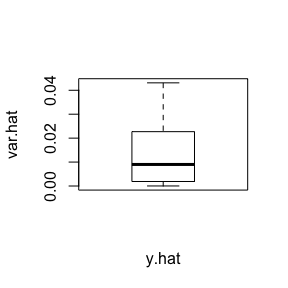It’s possible to look at the predicted probability of the classes, instead of class names:

ustat = forest.varU(u_test_prob,fit)
head(ustat)
##        y.hat     var.hat
## 1 0.01122024 0.000380496
## 2 0.07850881 0.005919839
## 3 0.22535333 0.019956682
## 4 0.13462405 0.006085724
## 5 0.11608048 0.006676548
## 6 0.32174524 0.013877763
plot(ustat)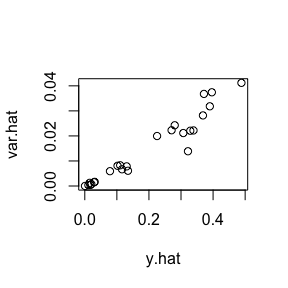Now we look at the training set (Case (1)), both class names and probabilities:

ustat = forest.varU(fit$predictedAll,fit) head(ustat) ## y.hat var.hat ## 1 absent 6.991374e-03 ## 2 absent 8.465584e-05 ## 3 absent 2.979472e-04 ## 4 absent 3.790655e-02 ## 5 absent 5.559198e-02 ## 6 present 2.397472e-02 plot(ustat)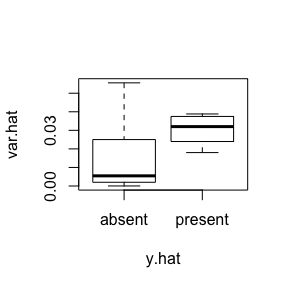ustat = forest.varU(fit$predictedProb,fit)
head(ustat)
##         y.hat      var.hat
## 1 0.080050476 0.0058511784
## 2 0.005188571 0.0001036628
## 3 0.006505476 0.0003087007
## 4 0.364473333 0.0367705552
## 5 0.390675000 0.0522782401
## 6 0.705473333 0.0246707625
plot(ustat)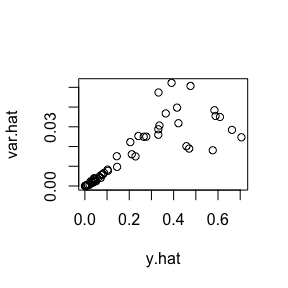### Infinitesimal jackknife based variance estimate

Again we can compare to the infinitesimal jackknife: (looks like there is a bug in the randomForestCI code (infinitesimalJackknife.R; line 144-145) in variance estimates for classification, so the code below is commented out until that package is fixed)

#rf = randomForest(x, y, keep.inbag = TRUE)
#ij = randomForestInfJack(rf, x, calibrate = TRUE)
#plot(ij)

Again we run the infinitesimal jackknife code in the randomForestCI package on our forest with sampling with replacement:

fit = forest(xtrain,ytrain,var.type="infjack",ntree=5000)
ij2_train_oob = fit$predicted # Case (1) ij2 = forest.varIJ(fit$predictedAll,fit)
head(ij2)
##    y.hat      var.hat
## 1 1.0662 0.0058837190
## 2 1.0000 0.0007481407
## 3 1.0000 0.0007481407
## 4 1.2410 0.0598847631
## 5 1.6526 0.0926676268
## 6 1.8816 0.0165616758
plot(ij2)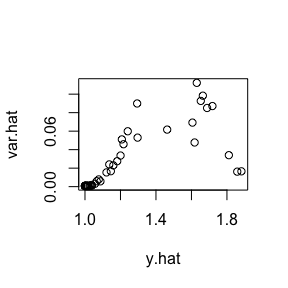### Compare to randomForest package

We end by comparing our forest’s predictions to that of the randomForest package

rf = randomForest(xtrain,ytrain,keep.forest=TRUE,keep.inbag=TRUE,replace=TRUE,ntree=5000)
rf_train_oob = rf\$predicted
table(ij2_train_oob,rf_train_oob)
##              rf_train_oob
## ij2_train_oob absent present
##       absent      46       2
##       present      2       6

## Implementation Notes

• Data with non-response, or classification with more than 2 categories is not yet supported. Contact us if you are eager for these!

• Categorical predictors are currently converted to their numeric equivalents, not made into indicator variables. This feature is pending.

• Like the randomForest package, the splitting criterion for regression is mean squared error, and gini impurity for binary classification.

## Mathematical Notes

• Minimizing gini impurity in binary classification is equivalent to minimizing mean squared error. For example, taking $$Y\in\{0,1\}$$, it can be shown that MSE = $$\frac{1}{2}$$ Gini impurity.

## Some Intuition (to be fleshed out)

In a foundational paper, Breiman (2001) showed that the variance of a random forest’s predictions is determined by two factors: the variance of each tree in the forest, and the correlation between trees. Intuitively, having trees that are certain about their predictions and less correlated with each other means the forest is reasonably certain .

One of the parameters driving correlation between individual trees is how many features they share - the higher the proportion of features each tree is allowed to see, the higher the probability that different trees have features in common. However, this parameter also affects the variance of an individual tree, as the less features a tree is allowed to see, the more uncertain its predictions.